# Multiplying fractions

## Multiplying fractions

### Multiplication fractions for a natural number

To multiply a fraction by a number, the numerator need to multiply the number and denominator remain unchanged.

### Example of multiplying fractions for a natural number

Example 1: Find the product of the fraction on an integer (multiply a fraction by a natural number):for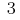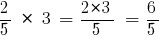Answer: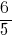### Multiplying fractions with different denominators

To add two fractions with different denominators, you need to:

### Example multiplication of fractions with different denominators

Example 2: Multiply two fractions with different denominators:and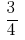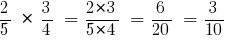Answer: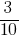### Multiplication of mixed numbers

To multiply two mixed numbers, you need to:

### Examples of adding mixed numbers

Example 3: Multiply the common fraction by a mixed number: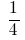and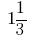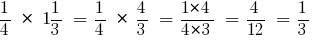Answer: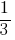Example 4 Multiply mixed number by a natural number: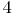and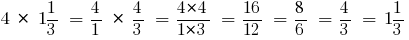Answer: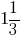Example 5: Multiply mixed numbers: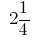and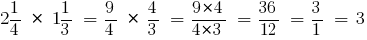Answer: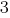## Multiplying decimals

To multiply two decimals, you should:

### Examples of multiplying decimals

Example 1: Multiply fractions: 13,2 and 0.2.

After completing the multiplication, ignoring the commas, we get: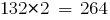.

Separate decimal point right as many digits as is the decimal point in both factors together, that is two digits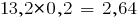.

Consider other examples of multiplication of decimal numbers:

Example 2:Example 3:### Multiplying decimals and integers

The product of decimals and natural numbers is called a sum of terms, each of which is equal to the given decimal, and the number of summands is equal to this positive integer.

Tomultiply a decimal fraction by a natural number, you need to:

• multiply it by that number, ignoring the decimal point;
• in the resulting product to separate the decimal point as many digits to the right, how many of them separated by a comma in decimal fractions.

### Multiplying decimals by 10, 100, 1000, etc.

Tomultiply a decimal fraction by 10,100,1000,etc., should:

• in this fraction it is necessary to move the decimal point that many digits to the right, how many zeros is in the multiplier after one.

Example 4: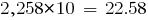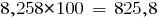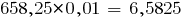If the result is fewer digits than necessary to separate with a comma, ahead write zero or several zeros.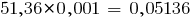Tags:
Chapter:
Versions in other languages:
Share with friends: